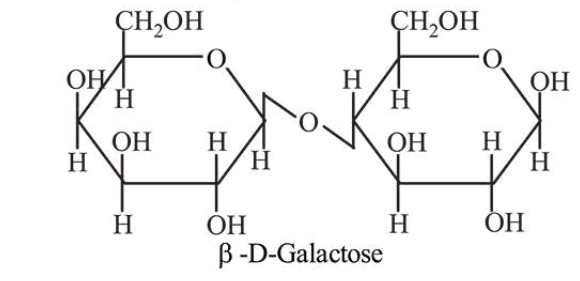# Which one of the following statements is not true?Question:

Which one of the following statements is not true?

1. Lactose contains $\alpha$-glycosidic linkage between $\mathrm{C}_{1}$ of galactose and $\mathrm{C}_{4}$ of glucose.

2. Lactose is a reducing sugar and it gives Fehling's test.

3. Lactose $\left(\mathrm{C}_{11} \mathrm{H}_{22} \mathrm{O}_{11}\right)$ is a disaccharide and it contains 8 hydroxyl groups.

4. On acid hydrolysis, lactose gives one molecule of $D(+)$-glucose and one molecule of $D(+)$-galactose

Correct Option: 1

Solution:

Lactose contains $\beta$-glycosidic linkage between $C_{1}$ of galactose and $C_{4}$ of glucose.